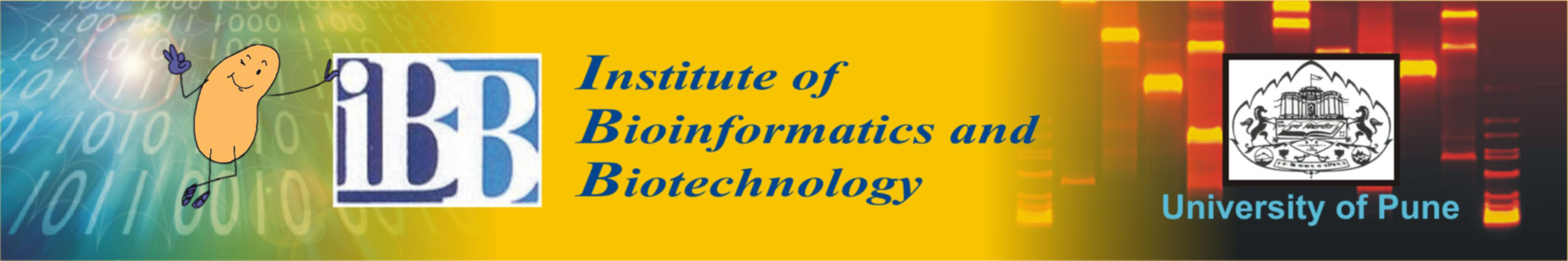# Team:IBB Pune/ModelingModelling

The aim of the modelling aspect of our project is to develop simulations that would enable us to predict the behavior of the export tag with the Snowdrift Game as the backdrop.

Project 1- A Model Of The Snowdrift Game

This is a Model of the Snowdrift game. This model assumes use of two different strains as co-operators and defectors.

Co-operators constitutively secrete/produce b-gal. Defectors cannot secrete/produce B-gal.

This model is for a Single iteration of the experiment i.e. the culture is initiated in shaker flask and allowed to grow. In order to find ESS or stable equilibria, we will have to repeatedly subculture the cells under similar conditions, by replacing the medium or supplying fresh medium. In such a setup, the input for the next cycle of experiments will be the output of the previous cycle of experiments.

Assumptions

At time t=0;

• There are 'k' co-operators and 'N-k' defectors
• Medium contains 'L' mg/ml of lactose, glucose conc. (g) = 0
• Culture is well mixed.
• Extracellular Enzyme conc (Ec) = 0 units/ml

Artificial assumptions

• Glucose is consumed by all cells. Galactose is also consumed at the same rate Gc mg/cell/min/ml.

• The metabolic benefit due to glucose and galactose is same.So effectively each lactose molecule gives rise to 2 glucose molecules

• There is no intracellular lactose metabolism (only extracellular).

• There is no lag in enzyme production and secretion.

• Rate of degradation of enzyme is zero

Model

In this Model,

1. Each co-operator secretes 'B' units of enzyme/ min and pays cost of 'c'/min

2. These molecules diffuse freely through the medium

3. They convert Lactose to Glucose + Galactose given by

```g = (k2)*(Ec)*(L) mg/ml/min	                       .... (1)
```

4. Absorbed glucose confers growth rate advantage of r

```r = (R)*(g)*(Gc)                                      .... (2)
```

After each min, the population of co-operators and defectors is updated depending upon their respective growth rates.

```D(t) = D(t-1)+ r * D(t-1)                             .... (3)     Defector population (t)
k(t) = k(t-1) + (r-c) * k(t-1)                        .... (4)     Co-operator population (t)
```

The Lactose that remains at time 't' is given by:

```L = L - L * Ec * k2                                   .... (5)
```

The net Glucose present in the medium at time 't' is given by:

```G = G +(( 2* L* Ec* k2)-( N * Gc)                     .... (6)
```

Results